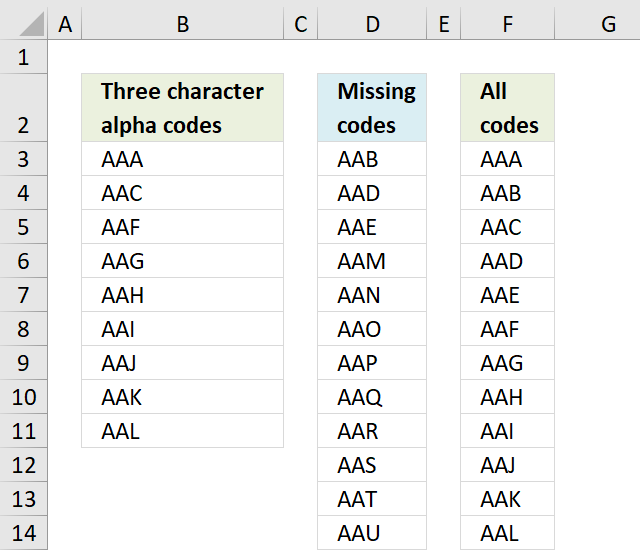Author: Oscar Cronquist Article last updated on November 29, 2018This blog article answers a comment in this blog article: Identify missing values in two columns using excel formula

Question: I need to do exactly the same thing, but with three character alpha codes instead of numbers. Can anyone help?

There are 26*26*26 = 17,576 different alpha codes beginning with AAA and ending with ZZZ. Column F contains all 17,576 alpha codes.

The formula in cell D3 compares the values in column B with the values in column F and extracts only those who are missing in column B.

Array formula in cell D3:

=INDEX(\$F\$3:\$F\$17578, SMALL(IF(COUNTIF(\$B\$3:\$B\$11, \$F\$3:\$F\$17578)=0, MATCH(ROW(\$F\$3:\$F\$17578), ROW(\$F\$3:\$F\$17578)), ""), ROWS(\$A\$1:A1)))

Explaining array formula in cell D3

Step 1 - Identify missing values

The COUNTIF function counts values based on a condition or criteria, in this case, it counts values in \$F\$3:\$F\$17578 based on \$B\$3:\$B\$11.

A 0 (zero) indicates a missing value.

COUNTIF(\$B\$3:\$B\$11, \$F\$3:\$F\$17578)=0

becomes

{0;1;0;1;1;0;0;0;0;0;0;0 ... 0}=0

and returns

{TRUE; FALSE; TRUE; FALSE; FALSE; TRUE; TRUE; TRUE; TRUE; TRUE; TRUE; TRUE}

Step 2 - Replace TRUE with corresponding row number

The IF function returns the corresponding row number if boolean value is TRUE. FALSE returns "" (nothing).

IF(COUNTIF(\$B\$3:\$B\$11, \$F\$3:\$F\$17578)=0, MATCH(ROW(\$F\$3:\$F\$17578), ROW(\$F\$3:\$F\$17578)), "")

becomes

IF({TRUE; FALSE; TRUE; FALSE; FALSE; TRUE; TRUE; TRUE; TRUE; TRUE; TRUE; TRUE}, MATCH(ROW(\$F\$3:\$F\$17578), ROW(\$F\$3:\$F\$17578)), "")

and returns

{"";2;"";4;5;"";"";"";"";"";"" ... ""}

Step 3 - Extract row number

The SMALL function returns the k-th smallest number in a cell range or array. SMALL(array, k)

SMALL(IF(COUNTIF(\$B\$3:\$B\$11, \$F\$3:\$F\$17578)=0, MATCH(ROW(\$F\$3:\$F\$17578), ROW(\$F\$3:\$F\$17578)), ""), ROWS(\$A\$1:A1))

becomes

SMALL({"";2;"";4;5;"";"";"";"";"";"" ... ""}, ROWS(\$A\$1:A1))

The ROWS function returns the number of rows in a cell reference, in this case the cell reference expands as the cell is copied to cells below.

SMALL({"";2;"";4;5;"";"";"";"";"";"" ... ""}, ROWS(\$A\$1:A1))

becomes

SMALL({"";2;"";4;5;"";"";"";"";"";"" ... ""}, 1)

and returns 2.

Step 4 - Return value

The INDEX function returns a value based on a row and column number. The column number is not needed here.

INDEX(\$F\$3:\$F\$17578, SMALL(IF(COUNTIF(\$B\$3:\$B\$11, \$F\$3:\$F\$17578)=0, MATCH(ROW(\$F\$3:\$F\$17578), ROW(\$F\$3:\$F\$17578)), ""), ROWS(\$A\$1:A1)))

becomes

INDEX(\$F\$3:\$F\$17578, 2)

and returns "AAB" in cell D3.

Get excel *.xlsm file

I made all the alpha codes using a UDF, you don't need the UDF to extract missing alpha codes so you can disable macros for this workbook if you like.

Three character alpha code.xlsm